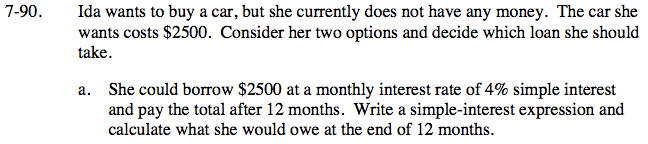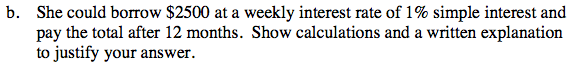Home > CC2 > Chapter 7 > Lesson 7.1.8 > Problem7-90

7-90.
1. Ida wants to buy a car, but she currently does not have any money. The car she wants costs $2500. Consider her two options and decide which loan she should take. Homework Help ✎ 1. She could borrow$2500 at a monthly interest rate of 4% simple interest and pay the total after 12 months. Write a simple-interest expression and calculate what she would owe at the end of 12 months.

2. She could borrow $2500 at a weekly interest rate of 1% simple interest and pay the total after 12 months. Show calculations and a written explanation to justify your answer.Use the equation for simple interest from the Math Notes box in this lesson. Interest = Prt where: P = Principal r = rate t = time Interest = ($2500)(0.04)(12 months)
($2500)(0.04) =$100 dollars per month in interest
($100)(12 months) =$1200 interest over 12 months

How much total money does Ida owe under this option if her borrowed amount is $2500 and her interest would be$1200?Use the same interest formula from part (a).
Interest = (principal)(interest rate)(weeks in a year)

There are 52 weeks in a year.

Ida owes \$3800 with this option. Be sure to show how to get this answer and explain which one of the two loan options is better and why.